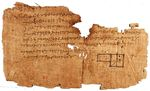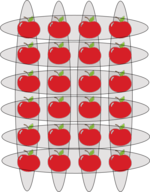# Why does math work?

by Daniel RanardMath works pretty well. We can count apples and oranges; we can scribble equations and then launch a rocket that lands gently upright. When an argument is indisputable, we colloquially say “do the math,” and we speak of events that will happen with “mathematical certainty.” Math works so well that you're forced to wonder: why, and what does it mean about our world? I won't fully answer these questions, but I'll offer a few perspectives.

You don't need to know much math to see it works. Say you go apple-picking with a friend; you count 12 as you pick them, and your friend counts 19 of her own. How many apples are in the basket? Maybe you crunch the numbers on a scrap of paper, just to be sure. You manipulate symbols on a page, and afterward you make a claim about reality: you know how many apples you would count if you pulled them out.But was that math or just common sense? If you're not impressed by addition, let's try multiplication. I suspect many of us encounter our first real mathematical “theorem” when we learn that A times B is B times A. As Euclid wrote circa 300 BC, “If two numbers multiplied by one another [in different orders] make certain numbers, then the numbers so produced equal one another.” This fact may be so familiar you forget its meaning: 4 x 6 = 6 x 4, or rather 6 + 6 + 6 + 6 = 4 + 4 + 4 + 4 + 4 + 4. It may be obvious, but a curious child would still ask, why? The equation demands proof, much like the Pythagorean Theorem. Euclid gave a proof in Book VII, Proposition 16 of the Elements. And though he proved an abstract fact using abstract symbols, the world seems to obey this arithmetic rule: if you have four groups of six apples, Euclid predicts you can always rearrange them into six groups of four.

Maybe it's no surprise we can use arithmetic to make these predictions. But what about the success of more sophisticated math and physics?

Euclid's Elements was full of theorems about numbers and geometry, most of them less obvious than the one above. He was not the first in the ancient world to write proofs, but with his axioms, definitions, and careful deductions, he was the most prolific and systematic. He collected others' proofs into one place and wrote many of his own. (In other words, he wrote a textbook. In fact, it was widely used for two thousand years.) And so mathematics continued onward, with new definitions and theorems filling whole libraries of books.

The laws of physics are formulated in this esoteric language. Sometimes, mathematicians even invent a new field of math before it's found to describe nature. For instance, “group theory” is a branch of mathematics pioneered in the 19th century. Physicists scrambled to learn it 100 years later when they realized that the fundamental particles are actually arranged according to certain patterns of these groups. Schrodinger famously complained about the use of group theory, the gruppenpest. But nature had its way, and now group theory is a cornerstone of modern physics.

The seeming mathematical nature of the world has long haunted specialists. Einstein asked, “How can it be that mathematics, being after all a product of human thought which is independent of experience, is so admirably appropriate to the objects of reality?” The physicist Eugene Wigner made the question famous in his 1960 essay, “The Unreasonable Effectiveness of Mathematics in the Natural Sciences.”

Wigner had in mind more advanced mathematics, but let's start with the question of arithmetic. It may seem significant that we can use arithmetic to describe the world, but we should ask: could it have been any other way? Is it conceivable to put 12 apples in a basket, then put in 19 more, but not count 31 when we take them out? Can we conceive of a world that defies all arithmetic analysis? We might imagine that even if normal arithmetic failed, a sensible world would exhibit some regularity, some patterns, and these patterns could then be formulated as precise rules, written in an unfamiliar framework that we would nonetheless call mathematics. However, I see no reason why the world should function according to any precise rules at all. We can imagine a world less predictable and more wild, where apples do as they please, and where you never know exactly what you'll see when you turn around. It's a world we experience in dreams. Borges writes about a similar place in his story Tlön, Uqbar, Orbis Tertius. “There are no sciences on Tlön, not even reasoning… The metaphysicians of Tlön do not seek for the truth or even for verisimilitude, but rather for the astounding.” Science may be possible in Tlön, but if so, its inhabitants are unaware.

We do not live in Tlön-ish dreamworld. So there's something special about our world, with its stable quantities of apples. What's more, the world exhibits consistent patterns susceptible to scientific analysis and prediction: Halley's comet will come every 75 years; Diet Coke and Mentos will always explode on contact. But I'm not just asking why science works. I'm asking why advanced math is so useful, why abstract equations in dusty libraries have come to describe everything from arrangements of apples to the trajectories of electrons.

A common answer is that maybe math doesn't work that well, and we just use it when it does. Bertrand Russell put it well: “Physics is mathematical not because we know so much about the physical world, but because we know so little; it is only its mathematical properties that we can discover.” Sure, we can use advanced mathematics to describe tiny particles, or the bending of spacetime under gravity. But what about… everything else that happens? Physicists seek out phenomena they can understand with careful experiments using immaculate equipment and precisely controlled conditions – but most of nature doesn't arrive that way. There are simple equations that predict the collisions of electrons, but we have no such equations to predict what people say, or how leopards move or mountains form. The mathematician Gelfand played off the title of Wigner's essay, writing about “the unreasonable ineffectiveness of mathematics in biology.” He's right: biology is a science, but not a precisely mathematical science. Math is only useful when it's useful.

It's true that math doesn't often yield precise predictions about biology, or indeed almost anything but physics. Still, I don't think this answer is satisfactory. It downplays a powerful fact: all matter we know is composed of small parts whose behavior is precisely described by mathematics. Without being reductionist – “a human being is just particles and equations” – we can still accept the significance here. Most physicists believe that if you gathered enough data and built a large enough computer (and that's a big, philosophically fraught “if”), you could simulate any situation from daily life, with predictions confounded only by quantum randomness. In fact, we already know the equations you would need. It appears our daily life is governed by these sophisticated equations, whether or not we have the data and computing power to solve them. And sure, maybe physicists are wrong. But even if God, Gods, or the free will of the human soul were to occasionally intervene, you would have to admit that the microscopic constituents of matter seem to follow these equations whenever we're watching.

To me, the scope of mathematical application is astonishing, even while limited, and I cannot explain it. But however you feel about the scope of math, we are left with Einstein's question, a different question. If mathematics is a product of pure thought, how do mathematicians develop theories that predate their use in physics? I already mentioned how the particle physics of the 20th century is largely built on group theory, a subject developed 100 years earlier, previously obscure to physicists. Another branch of mathematics developed in the 19th century was non-Euclidean geometry, the study of curved spaces. In the 1800s, the character Ivan in Dostoevsky's The Brothers Karamazov speaks of the absurdity and abstraction of non-Euclidean geometry, a subject which appears ungrounded in reality: “If God exists and if He really did create the world, then, as we all know, He created it according to the geometry of Euclid and the human mind with the conception of only three dimensions in space. Yet there have been and still are geometricians and philosophers, and even some of the most distinguished, who [nonetheless study non-Euclidean geometry].” Decades later, non-Euclidean geometry proved to be the crucial ingredient in Einstein's theory of relativity, describing the way that spacetime curves.

What accounts for these seeming miracles? To answer this question well would probably require a philosophy of mathematics – an account of where math comes from, and what mathematical truth is. But we can attempt some answers without a full-fledged philosophy. One simple response is that math is not “a product of human thought which is independent of experience,” despite Einstein's initial suggestion. (A closer reading of Einstein indicates he would agree.) For one, our minds are born of this world, shaped by millennia of evolution and interaction with the environment. Our thinking habits are in tune with the world. Maybe we acquired our specific arithmetic notions by observing the rearrangement of apples. Moreover, math and physics have been in communication for centuries, and they were not always perceived as distinct fields. “Geo-metry” began with measuring the earth, before it was codified into abstract definitions and symbols by Euclid and others. The new, non-Euclidean geometry of general relativity was developed decades before the physics, but it was partially inspired by the observation of curved surfaces like spheres. Should we be so surprised that spacetime itself turned out to be slightly curved, analogous to the way in which spheres curve?

Another possible explanation is that mathematicians simply invent a lot of math. Not all of it becomes central to our theories of nature, but we mainly notice when the math works. For instance, group theory is not the only abstraction of modern mathematics, but it just happens to be one of the most useful tools for classifying different types of particles. Or maybe particle physics could be re-formulated with a new branch of mathematics entirely, but physicists just used the math that was already available.

Each of these answers rings true, and to some they are totally satisfying. Mathematics is certainly inspired by observations of the physical world, and it is often developed for the express purpose of describing physics. But in mathematics, there are always infinite directions in which a field could grow. There's an overwhelming number of mathematical statements that one might try to prove. Which patch of the mathematical landscape do you choose to explore? Mathematicians say they are often guided by a sense of beauty and elegance, without regard for practical applications. Yet the mathematical objects they create sometimes describe the physical world with near-perfect fit. In fact, even physicists sometimes develop theories according to their sense of beauty and intuitive “rightness,” before these theories are confirmed by evidence. How do they do it? Probably the aesthetic of the physicist and mathematician is again born of the world itself, a product of experience and learned intuition.

Despite these explanations, I cannot convince myself that the striking success of mathematics is not a small miracle. So I will end by stealing Wigner's line: this miracle is “a wonderful gift which we neither understand nor deserve.”